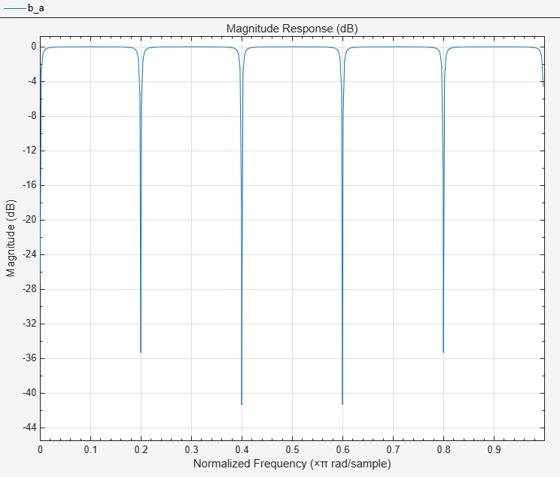# iircomb

IIR comb notch or peak filter

## Syntax

```[num,den] = iircomb(n,bw) [num,den] = iircomb(n,bw,ab) [num,den] = iircomb(...,'type') ```

## Description

`[num,den] = iircomb(n,bw)` returns a digital notching filter with order `n` and with the width of the filter notch at -3 dB set to `bw`, the filter bandwidth. The filter order must be a positive integer. `n` also defines the number of notches in the filter across the frequency range from 0 to 2π — the number of notches equals `n`+1.

For the notching filter, the transfer function takes the form

`$H\left(z\right)=b\frac{1-{z}^{-n}}{1-\alpha {z}^{-n}}$`

where α and b are the positive scalars and n is the filter order or the number of notches in the filter minus 1.

The quality factor (Q factor) q for the filter is related to the filter bandwidth by q = ω0/bw where ω0 is the frequency to remove from the signal.

`[num,den] = iircomb(n,bw,ab)` returns a digital notching filter whose bandwidth, `bw`, is specified at a level of -`ab` decibels. Including the optional input argument `ab` lets you specify the magnitude response bandwidth at a level that is not the default -3 dB point, such as -6 dB or 0 dB.

`[num,den] = iircomb(...,'type')` returns a digital filter of the specified type. The input argument `type` can be either

• `'notch'` to design an IIR notch filter. Notch filters attenuate the response at the specified frequencies. This is the default type. When you omit the `type` input argument, `iircomb` returns a notch filter.

• `'peak'` to design an IIR peaking filter. Peaking filters boost the signal at the specified frequencies.

The transfer function for peaking filters is

`$H\left(z\right)=b\frac{1-{z}^{-n}}{1+a{z}^{-n}}$`

## Examples

collapse all

Design and plot an IIR notch filter with 11 notches (equal to filter order plus 1) that removes a 60 Hz tone (f0) from a signal at 600 Hz(fs). For this example, set the Q factor for the filter to 35 and use it to specify the filter bandwidth.

```fs = 600; fo = 60; q = 35; bw = (fo/(fs/2))/q; [b,a] = iircomb(fs/fo,bw,'notch'); % Note type flag 'notch' fvtool(b,a);```Using the Filter Visualization Tool (FVTool) generates the following plot showing the filter notches. Note the notches are evenly spaced and one falls at exactly 60 Hz.